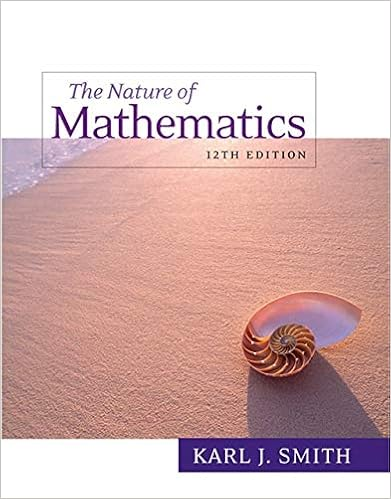# Math 2070u midterm march 1st 2018 page 7 of 11 4 7

• Test Prep
• 11
• 100% (7) 7 out of 7 people found this document helpful

This preview shows page 7 - 11 out of 11 pages.

##### We have textbook solutions for you!
The document you are viewing contains questions related to this textbook.The document you are viewing contains questions related to this textbook.
Chapter 17 / Exercise 60
Nature of Mathematics
SmithExpert Verified
MATH 2070U Midterm March 1st, 2018 Page 7 of 11 4. [7 marks] Let the matrix A be given by A = 1 2 0 3 1 1 2 1 - 1 . Compute the LU factorisation PA = LU using partial pivoting, where P is a permutation matrix, L is unit lower triangular, and U is upper triangular. Show your work! Produced with a Trial Version of PDF Annotator -
##### We have textbook solutions for you!
The document you are viewing contains questions related to this textbook.The document you are viewing contains questions related to this textbook.
Chapter 17 / Exercise 60
Nature of Mathematics
SmithExpert Verified
MATH 2070U Midterm March 1st, 2018 Page 8 of 11 This page has been left blank on purpose. Use it for scratch work. Nothing on this page will be graded.
MATH 2070U Midterm March 1st, 2018 Page 9 of 11 5. Given f ( x ) = x 3 + 4 x 2 - 10, the equation f ( x ) = 0 has a unique root in [ 1, 1.5 ] . With simple algebraic manipulations we can show that both x = g 1 ( x ) : = 1 2 ( 10 - x 3 ) 1/2 and x = g 2 ( x ) : = ( 10 4 + x ) 1/2 change the given equation to the fixed point iteration. Knowing that g 0 1 ( x ) = - 3 4 x 2 ( 10 - x 3 ) - 1/2 and g 0 2 ( x ) = - 5 10 ( 4 + x ) 3/2 , answer the following questions. (a) [ 1 / 2 mark] What is the value of g 0 1 ( 1.5 ) ? (a) (b) [ 1 / 2 mark] What is the value of | g 0 2 ( 1.5 ) | ? (b) (c) [2 marks] Based on your result for parts (a) and (b) which of the above fixed point iterations x = g 1 ( x ) or x = g 2 ( x ) will converge more rapidly? Justify your answer! (d) [4 marks] Based on your answer for part (c) choose the more efficient fixed point iteration and perform 3 steps of the iteration starting at x 0 = 1.5. Show your work! (e) [2 marks] The actual value of the root is x * = 1.3652 . Using the result of your computation in part (d) compute the relative error at the third iteration. Show your work! Produced with a Trial Version of PDF Annotator -
MATH 2070U Midterm March 1st, 2018 Page 10 of 11
•••